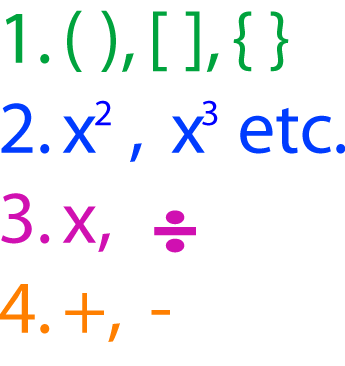# Definition of Order of OperationsThe order of operations provides a list of rules that tell you the order in which to apply the operations that appear in a mathematical expression.

These rules are:

1. Do everything inside brackets first (working from the innermost brackets outwards).
2. Next, take powers of things.
3. Next, multiply and divide, working from left to right when there is division involved.

For example,

\begin{align*} 8 - (3 + 2) \times 6 + 4 \div 2 &= 8 - 5 \times 6 + 4 \div 2\\ &= 8 - 30 + 2\\ &= -20 \end{align*}

### Description

The aim of this dictionary is to provide definitions to common mathematical terms. Students learn a new math skill every week at school, sometimes just before they start a new skill, if they want to look at what a specific term means, this is where this dictionary will become handy and a go-to guide for a student.

### Audience

Year 1 to Year 12 students

### Learning Objectives

Learn common math terms starting with letter O

Author: Subject Coach
You must be logged in as Student to ask a Question.